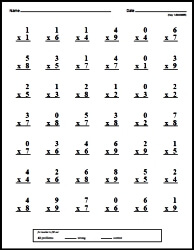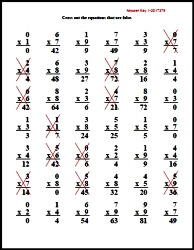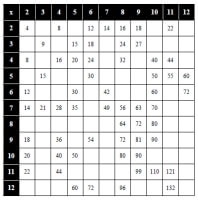edHelper.com Math Worksheets Multiplication Worksheets 2nd Grade MultiplicationWorksheets, Lessons, and Printables

Math Facts

Quick Math FactsMake Quick Math Facts PrintableMultiplication Table and ChartMultiplication Table and Chart

Introduction to Multiplication (Part 1)
Multiplication Concepts (with graphics of pictures)
Write the sum and product
Write the addition and multiplication fact
Write the sum and product (smaller graphics)
Write the addition and multiplication fact (smaller graphics)
Multiplication with Rows (dot graphics)
Multiply with 2
Multiply with 3
Multiply with 4
Multiply with 5
Multiply with 6
Mixed
Write the sum and then write the product
Multiply with 2
Multiply with 3
Multiply with 4
Multiply with 5
Multiply with 6
Multiply with 7
Multiply with 8
Multiply with 9
Multiply with 10
Mixed
Addition and Multiplication: Fill in the missing numbers
Multiply with 2
Multiply with 3
Multiply with 4
Multiply with 5
Multiply with 6
Multiply with 7
Multiply with 8
Multiply with 9
Multiply with 10
Mixed
Addition and Multiplication: Comparison
Comparison
Introduction to Multiplication: Mixed Review
Introduction to Multiplication Mixed Review

Multiplication and Division
Fill in the quotient
Fill in the missing number
Fill in the missing number

Multiplication

Have a suggestion or would like to leave feedback?